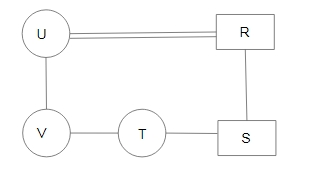Question 14

# $$A + B$$ means ‘A is the father of B’:$$A - B$$ means ‘A is the sister of B’;$$A \times B$$ means ‘A is the brother of B’;$$A \div B$$ means ‘A is the daughter of B’.If, $$R + S \times T - V \div U$$, then how is S related to U?

Solution

In the diagram, Circle shows the female, square shows the male, vertical lines show the generation, single horizontal line shows the brother/sister and double horizontal lines show the couple.From the diagram,
'S' is the son of 'U'.
$$\therefore$$ The correct answer is option A.

• Free SSC Study Material - 18000 Questions
• 230+ SSC previous papers with solutions PDF